Disclaimer: This is an example of a student written essay.

Any scientific information contained within this essay should not be treated as fact, this content is to be used for educational purposes only and may contain factual inaccuracies or be out of date.

# Lab Report: Heat Capacity Ratio For Gases

 ✅ Paper Type: Free Essay ✅ Subject: Chemistry ✅ Wordcount: 1932 words ✅ Published: 26th Jan 2018

• Jekathjenani Ratnakumaran

Introduction:

Heat capacity of gases is the amount of heat required to increase the temperature of one mole of the gas through one degree Celsius at constant pressure or volume. Heat capacity at constant pressure (Cp), the volume will increase and heat capacity at constant volume (CV), the pressure will increase. The main physical properties of gases are compressed in the state, expand themselves to fill the entire containers, and thus obeys the second law of thermodynamics. It occupies more space, compare to liquids and solids. Gases has no fixed shape. The physical state of the gases can be defined by,

PV = nRT

P- pressure of the gas, V- Volume of the gas, T- Temperature of the gas, n- number of moles of the substance present on the gas and R- Gas constant.

The purpose of this study is to determine the value of the heat capacity ratio, γ = Cp/CV for giving gases such as argon, oxygen, nitrogen and nitrous oxide using adiabatic expansion. This experiment is mainly based on measuring the pressure of the gases. Pressure can be measured by the force applied by the collision of the molecules or atoms with the surface or the walls of the tube. Pressure is measured in psi (Pounds per Square Inch) unit using manometer (P.Atkins et al, 2008). At room temperature, nitrogen is a colorless diatomic molecule of gas. It occupied 78.09% of earth’s atmosphere. Nitrogen occurs in all living organisms and about 3% of nitrogen are available in human body. Similarly, oxygen is a colorless, diatomic molecules and occupied about 20.95% earth’s atmosphere. It’s a highly reactive organic element and also it plays an important role in the respiration mechanism. Argon is another important gas, also called as inert gas as it is stable. It occupied about 0.93% of earth’s atmosphere and its colorless, nontoxic element. Nitrous oxide is a colorless, nonflammable gas and also known as ‘laughing gas’. It also takes place in the earth’s atmosphere as it is produced by the bacteria in the soil and ocean. Adiabatic expansion is the process which takes place with no transfer of heat between the system and surroundings. The ratio, γ will be compared with the theoretical value. Using the adiabatic expansion, three states of gas such as before the expansion (P1), immediately after expansion (P2) and after returning to room temperature (P3) can be observed. Based on these values, the heat capacity ratio can be determined. The ratio of heat capacity can be calculated using the following formula,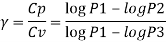γ – ratio of heat capacity

Cp- heat capacity at constant pressure

Cv- heat capacity at constant volume

Methods:

This experiment was conducted by following the procedure given in the laboratory manual by the department of chemistry, Trent University.

1. Ideal gas law,

PV = nRT

As the density, ρ = m/V, the density can be calculated using the above equation,

ρ = PM / RT

P = Pressure; M = Molar mass; R = gas constant; T = Temperature

For Nitrogen,

P = 14.476 psi = 1.018 kg/cm2

T = 22 0C = 295 K

M = 0.028 Kg /mol

R = 8.314 Jm3/Kmol = 84.784 x 10-6 m3 kg/cm2.K-1mol-1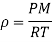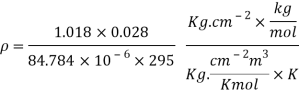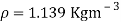Using the above calculation method, density of remaining gases was calculated.

For oxygen, ρ = 1.301 Kgm-3

For argon, ρ = 1.595 Kgm-3

For nitrous oxide, ρ = 1.790Kgm-3

1. PV = nRT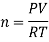P = 14.550 psi = 0.9900 atm

V = 30 L

R = 0.08206 Latm/Kmol

T = 295K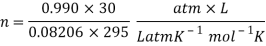n = 1.227 mol

Molecules = n x 6.022 x1023

= 7.389 x 1023 molecules the carboy holds under prevailing laboratory conditions.

1. For nitrogen,

Collision frequency of molecules of nitrogen can be calculated using the following formula,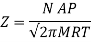N = 6.022 x 1023/ mol

A = 0.60 m2

P = 0.985 atm = 99808.5066 pa

R = 8.314 J/K mol = 8.314 Kg m2 /s2 K mol

M = 28 g / mol = 0.028 Kg/mol

T = 295 K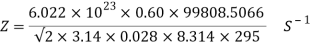Z = 1.736 x 1027 s-1

Results:

Table 1 represents the observations and reading of pressure obtained for the Argon, Oxygen, Nitrogen and Nitrous Oxide. The unit of pressure is recorded in psi.

 Gas Run P1 (psi) P2 (psi) P3 (psi) γ = (Cv/Cp) Mean Value of γ N2 1 13.652 14.552 14.332 1.313393 1.2815 N2 2 13.570 14.550 14.350 1.247655 N2 3 13.577 14.547 14.327 1.283416 O2 1 13.561 14.491 14.467 1.02563 1.0248 O2 2 13.554 14.494 14.470 1.025342 O2 3 13.524 14.494 14.471 1.023465 Ar 1 13.590 14.550 14.270 1.397982 1.4268 Ar 2 13.470 14.550 14.190 1.481127 Ar 3 13.430 14.550 14.220 1.401368 N2O 1 13.468 14.488 14.470 1.017324 1.0163 N2O 2 13.508 14.488 14.473 1.015012 N2O 3 13.379 14.499 14.480 1.016581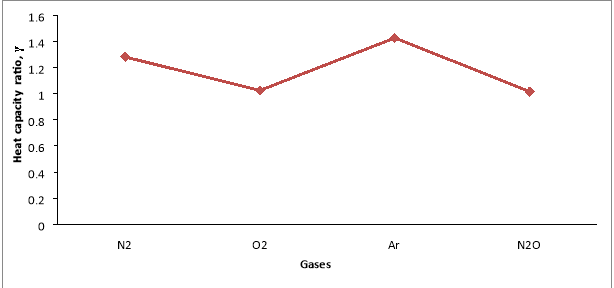Figure 1 represents the heat capacity ratio of the given gases: nitrogen, Oxygen, Argon and Nitrous Oxide.

Data Analysis & Calculations: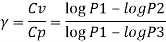For Nitrogen: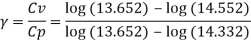γ1 = – 0.02773 / – 0.02111

= 1.3134

Mean value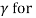Nitrogen,

γ = (γ1 + γ2 + γ3) / 3

= (1.3134 + 1.2476 + 1.2834) / 3

= 1.2814

Discussion:

The experiment was conducted with careful consideration to get the result as close as to the real value. However, due to the some experimental error involved in this experiment, the calculated values are not close to the literature value. The calculated heat capacity ratios for the given gases: nitrogen, oxygen, argon, and nitrous oxide are 1.2815, 1.0248, 1.4268, 1.0163 respectively. The literature heat capacity ratio values are 1.4000 (nitrogen), 1.6667 (argon), 1.4000 (oxygen) and 1.3100 (nitrous oxide) (P.Atkins et al, 2008). Figure 1 represents the heat capacity ratio of the given gases. Based on the graph shown on figure 1, heat capacity ratio of oxygen and nitrous oxide are lower than the nitrogen and argon. In comparison with the literature value, nitrogen and argon are closer to the calculated heat capacity value. However, the calculated percentage error is 8.46% and 14.4% respectively. In the case of oxygen and nitrous oxide, calculated value more deviated from the literature value and the calculated percentage error are 26.8% and 22.42% respectively. These differences between the calculated and literature value heat capacity ratio value occurred due to the experimental error. Equipments used in this experiment were used by the previous batch. As the experiment was conducted using the pre setup equipments, there might be an error in the flow of gases through the tubes. Due to these occurrences, there might be changes in the pressure. Since the flow rate of gases are in larger amount, it could have heavily affected the final results. Gas leak is one of the major concern which lead to decrease in pressure and affected the result. Moreover, the adjustment made at the flow rate of gases also might be lead to the error in the heat capacity value. As the digital manometer is open ended to the atmosphere, there might be an error due to the unstable atmospheric pressure. Furthermore, due to the weather condition (winter), temperature and pressure changes and might not favored to conduct the experiment.

Conclusion:

In conclusion, the heat capacity ratio of nitrogen, oxygen, argon and nitrous oxide were calculated using the adiabatic expansion. In order to get accuracy of result, the precision can be improved by more attention on the experimental procedure and handling the equipments. Also, accurate result can be made by observing the measurements such as notifying the pressure of gases and units in a proper manner.

References

1. K.Lunder et al (1999); Heat Capacity Ratios For Gases: The Sound Velocity Method, Physical Chemistry 301, Augustana College
2. P. Atkins and J. de Paula, Atkins’ Physical Chemistry, 8th ed., W. H.Freeman and Co., New York (2006).

View all

## DMCA / Removal Request

If you are the original writer of this essay and no longer wish to have your work published on UKEssays.com then please:

Related Services

Prices from

S\$203

Approximate costs for:

• 1000 words
• 7 day deliveryHumanity University

Dedicated to your worth and value as a human being!

Related Lectures